# Lecture 1 | AVL Trees & Splay Trees¶

Like

Like this

Like this!

## AVL 树¶

### 概念¶

height of a tree

#### AVL 树¶

AVL Tree

1. 一个空二叉树是一个 AVL 树；
2. 如果二叉树 $$T$$ 是一个 AVL 树，则其左右孩子树 $$T_l$$$$T_r$$ 也都应该是 AVL 树，且有 $$|h(T_l) - h(T_r)| \leq 1$$

Balance Factor

$BF(T_p) = h(T_l) - h(T_r)$

• 如果二叉树 $$T$$ 是一个 AVL 树，则其左右孩子树 $$T_l$$$$T_r$$ 也都应该是 AVL 树，且有 $$BF(T_p) \in \{0, \pm 1\}$$

Height of AVL Trees

graph TD;
A(("Root"))
B[/"Left Subtree"\]
C[/"Right Subtree"\]
A === B
A === C

$n_h = \left\{ \begin{array}{l} 1 & (h = 0) \\ 2 & (h = 1) \\ n_{h-1} + n_{h-2} + 1 & (h > 1) \end{array} \right.$

$F_i = \left\{ \begin{array}{l} 1 & (i = 1) \\ 1 & (i = 2) \\ F_{i-1} + F_{i-2} & (i > 2) \end{array} \right.$

\begin{aligned} F_n &= \frac{1}{\sqrt{5}} \left( \left( \frac{1 + \sqrt{5}}{2} \right)^n - \left( \frac{1 - \sqrt{5}}{2} \right)^n \right) \\ &\approx \frac{1}{\sqrt{5}} \left( \frac{1 + \sqrt{5}}{2} \right)^n \\ \log{(F_n)} &\approx n \end{aligned}

$$n_h + 1 \approx F_{h+2}$$，所以 $$h \approx \log{(n_h)}$$，也就是说 $$h \approx \log{N}$$

#### 视角¶

Trouble Maker & Trouble Finder

graph TD;
A(("8, BF=1"))
B(("4, BF=0"))
C(("9, BF=0"))
D(("2, BF=0"))
E(("6, BF=0"))
A === B
A === C
B === D
B === E

graph TD;
A(("8, BF=2"))
B(("4, BF=-1"))
C(("9, BF=0"))
D(("2, BF=0"))
E(("6, BF=1"))
F(("5, BF=0"))
A === B
A === C
B === D
B === E
E === F
E === NULL

### 操作¶

flowchart TD
A((("Trouble\nFinder")))
B((("Left\nChild")))
C(("Right\nChild"))
D(("L Left\nChild"))
DA[/"Left and Right Subtrees"\]
E(("L Right\nChild"))
EA[/"Left and Right Subtrees"\]
F(("R Left\nChild"))
FA[/"Left and Right Subtrees"\]
G(("R Right\nChild"))
GA[/"Left and Right Subtrees"\]
H(("Trouble\nMaker"))
A === B
A === C
B === D === DA === H
B === E === EA
C === F === FA
C === G === GA
flowchart TD
A((("Trouble\nFinder")))
B(("Left\nChild"))
C((("Right\nChild")))
D(("L Left\nChild"))
DA[/"Left and Right Subtrees"\]
E(("L Right\nChild"))
EA[/"Left and Right Subtrees"\]
F(("R Left\nChild"))
FA[/"Left and Right Subtrees"\]
G(("R Right\nChild"))
GA[/"Left and Right Subtrees"\]
H(("Trouble\nMaker"))
A === B
A === C
B === D === DA
B === E === EA
C === F === FA
C === G === GA === H
flowchart TD
A((("Trouble\nFinder")))
B((("Left\nChild")))
C(("Right\nChild"))
D(("L Left\nChild"))
DA[/"Left and Right Subtrees"\]
E((("L Right\nChild")))
EA[/"Left and Right Subtrees"\]
F(("R Left\nChild"))
FA[/"Left and Right Subtrees"\]
G(("R Right\nChild"))
GA[/"Left and Right Subtrees"\]
H(("Trouble\nMaker"))
A === B
A === C
B === D === DA
B === E === EA === H
C === F === FA
C === G === GA
flowchart TD
A((("Trouble\nFinder")))
B(("Left\nChild"))
C((("Right\nChild")))
D(("L Left\nChild"))
DA[/"Left and Right Subtrees"\]
E(("L Right\nChild"))
EA[/"Left and Right Subtrees"\]
F((("R Left\nChild")))
FA[/"Left and Right Subtrees"\]
G(("R Right\nChild"))
GA[/"Left and Right Subtrees"\]
H(("Trouble\nMaker"))
A === B
A === C
B === D === DA
B === E === EA
C === F === FA === H
C === G === GA

#### LL & RR¶

##### “旋转”视角¶

LLRR 这两种情况相对直观。由于它们的操作是对称的，所以我这里以 LL 为例。

    flowchart TD
A((("Trouble\nFinder")))
C[/"Right Subtree"\]

subgraph SA["New Left Subtree"]

subgraph SB["New L Left Subtree"]
D[/"L Left Subtree"\]
H(("Trouble\nMaker"))
end

B((("Left\nChild")))
E[/"L Right Subtree"\]
end

A === B
A === C
B === D === H
B === E

1. $$BF(\text{Trouble Finder}) = h(\text{New Left Subtree}) - h(\text{Right Subtree}) = 2$$
2. $$h(\text{New L Left Subtree}) - h(\text{L Right Subtree}) = 1$$
• 如果此差为 0，则不应当成为 Trouble Maker，若此差为 2，则 Left Child 应当为 Trouble Finder；

LL Single Rotation

    flowchart TD
A((("Trouble\nFinder")))
B((("Left\nChild")))
C[/"Right Subtree"\]
D[/"L Left Subtree"\]
H(("Trouble\nMaker"))
E[/"L Right Subtree"\]

A === B
A === C
B === D === H
B === E
    flowchart TD
A((("Trouble\nFinder")))
B((("Left\nChild")))
C[/"Right Subtree"\]
D[/"L Left Subtree"\]
E[/"L Right Subtree"\]
H(("Trouble\nMaker"))

A === B
A --- E
A === C
B === D === H
B === E
    flowchart TD
A((("Trouble\nFinder")))
B((("Left\nChild")))
C[/"Right Subtree"\]
D[/"L Left Subtree"\]
E[/"L Right Subtree"\]
H(("Trouble\nMaker"))

B === D === H
B --- E
B === A
A === E
A === C

    flowchart TD
A((("Trouble\nFinder")))
B((("Left\nChild")))
C[/"Right Subtree"\]
D[/"L Left Subtree"\]
E[/"L Right Subtree"\]
H(("Trouble\nMaker"))

B === D === H
B === A
A === E
A === C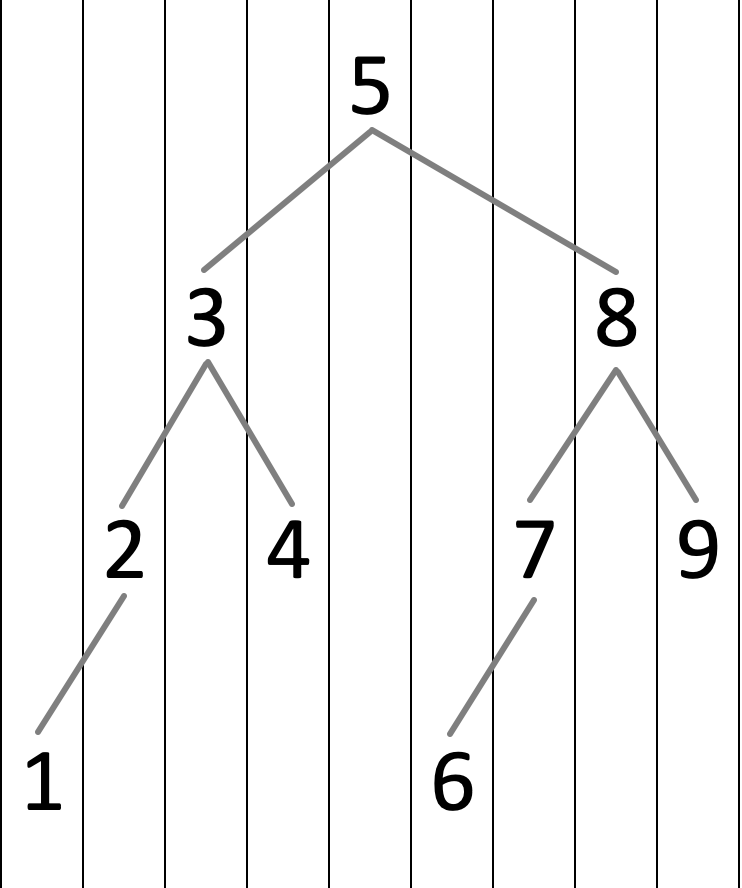##### “换根”视角¶

LL Single Rotation

    flowchart TD
A((("Trouble\nFinder")))
B((("Left\nChild")))
C[/"Right Subtree"\]
D[/"L Left Subtree"\]
H(("Trouble\nMaker"))
E[/"L Right Subtree"\]

A === B
A === C
B === D === H
B === E
    flowchart TD
A((("Trouble\nFinder")))
B((("Left\nChild")))
C[/"Right Subtree"\]
D[/"L Left Subtree"\]
H(("Trouble\nMaker"))
E[/"L Right Subtree"\]

A --- B
A === C
B === D === H
B === E
    flowchart TD
B((("Left\nChild")))
D[/"L Left Subtree"\]
E[/"L Right Subtree"\]
H(("Trouble\nMaker"))
A((("Trouble\nFinder")))
C[/"Right Subtree"\]

A === C
B === D === H
B === E
    flowchart TD
B((("Left\nChild")))
D[/"L Left Subtree"\]
E[/"L Right Subtree"\]
H(("Trouble\nMaker"))
A((("Trouble\nFinder")))
C[/"Right Subtree"\]

A === C
B === D === H
A === E
    flowchart TD
A((("Trouble\nFinder")))
B((("Left\nChild")))
C[/"Right Subtree"\]
D[/"L Left Subtree"\]
E[/"L Right Subtree"\]
H(("Trouble\nMaker"))

B === D === H
B === A
A === E
A === C


OK，你可能觉得这个解释对于 LL Single Rotation 来说显得十分多此一举，但是这都是为了给后面做的铺垫，虽然这个方法看起来繁琐冗余，但是已经足以解释原先的 Single Rotation 无法解释的 LR & RL 操作方法。

#### LR & RL¶

LR Rotation

    flowchart TD
A((("Trouble\nFinder")))
B((("Left\nChild")))
C[/"Right Subtree"\]
D(("L Left\nChild"))
DA[/"L Left Subtree"\]
H(("Trouble\nMaker"))
E((("L Right\nChild")))
EA[/"L Left Subtree"\]
EB[/"L Right Subtree"\]

A === B
A === C
B === D === DA
B === E === EA --- H
E === EB --- H
    flowchart TD
A((("Trouble\nFinder")))
B((("Left\nChild")))
C[/"Right Subtree"\]
D(("L Left\nChild"))
DA[/"L Left Subtree"\]
H(("Trouble\nMaker"))
E((("L Right\nChild")))
EA[/"L Left Subtree"\]
EB[/"L Right Subtree"\]

A --- B
A === C
B === D === DA
B --- E === EA --- H
E === EB --- H
    flowchart TD

B((("Left\nChild")))
D(("L Left\nChild"))
DA[/"L Left Subtree"\]
H(("Trouble\nMaker"))
E((("L Right\nChild")))
EA[/"L Left Subtree"\]
EB[/"L Right Subtree"\]
A((("Trouble\nFinder")))
C[/"Right Subtree"\]

A === C
B === D === DA
E === EA --- H
E === EB --- H
    flowchart TD

B((("Left\nChild")))
D(("L Left\nChild"))
DA[/"L Left Subtree"\]
H(("Trouble\nMaker"))
E((("L Right\nChild")))
EA[/"L Left Subtree"\]
EB[/"L Right Subtree"\]
A((("Trouble\nFinder")))
C[/"Right Subtree"\]

A === C
B === D === DA
E --- EA --- H
E --- EB --- H
    flowchart TD

D(("L Left\nChild"))
B((("Left\nChild")))
DA[/"L Left Subtree"\]
E((("L Right\nChild")))
EA[/"L Left Subtree"\]
EB[/"L Right Subtree"\]
H(("Trouble\nMaker"))
A((("Trouble\nFinder")))
C[/"Right Subtree"\]

E === B
E === A
B === D === DA
B === EA --- H
A === EB --- H
A === C

    flowchart TD
A((("Trouble\nFinder")))
B((("Left\nChild")))
C[/"Right Subtree"\]
D(("L Left\nChild"))
DA[/"L Left Subtree"\]
H(("Trouble\nMaker"))
E((("L Right\nChild")))
EA[/"L Left Subtree"\]
EB[/"L Right Subtree"\]

A === B
A === C
B === D === DA
B === E === EA --- H
E === EB --- H
    flowchart TD
A((("Trouble\nFinder")))
B((("Left\nChild")))
C[/"Right Subtree"\]
D(("L Left\nChild"))
DA[/"L Left Subtree"\]
H(("Trouble\nMaker"))
E((("L Right\nChild")))
EA[/"L Left Subtree"\]
EB[/"L Right Subtree"\]

A --- B
A --- E
A === C
B === D === DA
B --- E === EA --- H
E === EB --- H
    flowchart TD
A((("Trouble\nFinder")))
B((("Left\nChild")))
D(("L Left\nChild"))
DA[/"L Left Subtree"\]
H(("Trouble\nMaker"))
E((("L Right\nChild")))
EA[/"L Left Subtree"\]
EB[/"L Right Subtree"\]
C[/"Right Subtree"\]

A --- B
E --- B
E --- A
B === D === DA
B --- EA --- H
A --- EB --- H
A === C
    flowchart TD
A((("Trouble\nFinder")))
B((("Left\nChild")))
D(("L Left\nChild"))
DA[/"L Left Subtree"\]
H(("Trouble\nMaker"))
E((("L Right\nChild")))
EA[/"L Left Subtree"\]
EB[/"L Right Subtree"\]
C[/"Right Subtree"\]

E --- B
E --- A
B === D === DA
B --- EA --- H
A --- EB --- H
A === C

## Splay 树¶

Splay 树，即伸展树，想要解决的问题和 AVL 树类似，只不过 Splay 树希望达到的目标是在摊还(Amortized)复杂度$$O(\log N)$$的情况下完成大部分对点操作。

Splay 的核心思想就是，每当我们访问一个节点（比如查询某个点、插入某个点，甚至是删除某个点），我们就通过一系列操作将目标点转移到根部，形象上理解就是不断旋转整个树的构造，知道把点转到根部。

（没玩过可能听不太懂）不知道大家有没有玩过那种电子游戏，就是有好多转盘，相邻转盘之间有交界处，这些交界点上都会有圆球，当一个转盘旋转的时候，其圆周上的球体都会跟着这个圆周转动。通过不停转动相邻的圆盘，我们可以实现把某个位置的圆球转移到另外一个位置。而 Splay 差不多是这么个感觉。

### 基本操作¶

• 当我们访问到某个点 X 时：
• 如果 P 是根节点，则直接进行一次 Single Rotation，将 X 转到根节点；
• 如果 P 不是根节点：
• 当情况为 LR / RL 时，进行一次 LR Rotation 或 RL Rotation，我们称之为 zig-zag；
• 当情况为 LL / RR 时，进行两次 Single Rotation，使得 X、P、G 的顺序逆转，像跷跷板一样，我们称之为 zig-zig；
• 不断对 X 进行 Splay 操作，直到 X 成为根节点；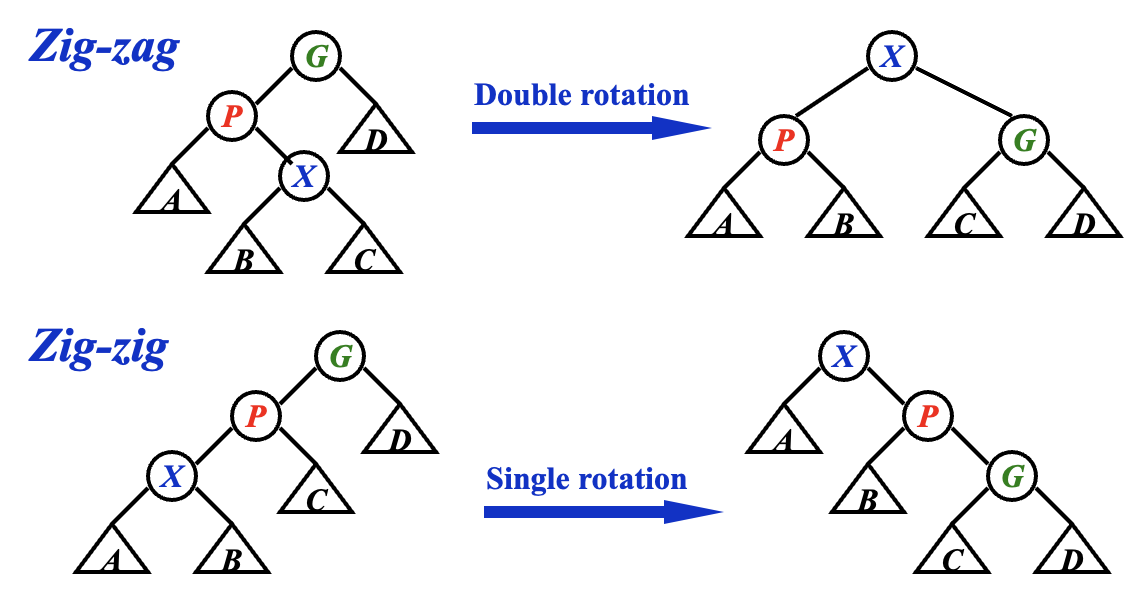Splaying not only moves the accessed node to the root, but also roughly halves the depth of most nodes on the path.

Find X

Remove X

Find Max

### 摊还分析¶

$T_{\text{rotate }x\text{ to root}} = T_{\text{zig}} + \sum{ {T_{\text{zig-zag}}}_i } + \sum{ {T_{\text{zig-zig}}}_i }$

• 开销大的操作应当倾向让势能降，开销小的操作应当倾向让势能升；
• 势能高倾向于让某些操作开销大，势能低倾向于让某些操作开销小；
• $$\Phi(final) > \Phi(initial)$$

\Phi(T) = \sum_{des \in T} \log Size(des) = \sum_{des \in T} Rank(des)\\ \begin{aligned} &\text{Where } des \text{ means the descendant of } T \text{,}\\ &\text{and } Size(des) \text{ means the number of the nodes of } des \text{.} \\ &\text{And we note } Rank(des) = \log Size(des) \approx Height(des) \text{.} \end{aligned}

Rank v.s. Height

$T_{\text{rotate }x\text{ to root}} = T_{\text{zig}} + \sum{ {T_{\text{zig-zag}}}_i } + \sum{ {T_{\text{zig-zig}}}_i }$

$\hat{c}_{\text{rotate }x\text{ to root}} = \hat{c}_{\text{zig}} + \sum{ {\hat{c}_{\text{zig-zag}}}_i } + \sum{ {\hat{c}_{\text{zig-zig}}}_i }$

#### zig¶

Amortized Analysis for Zig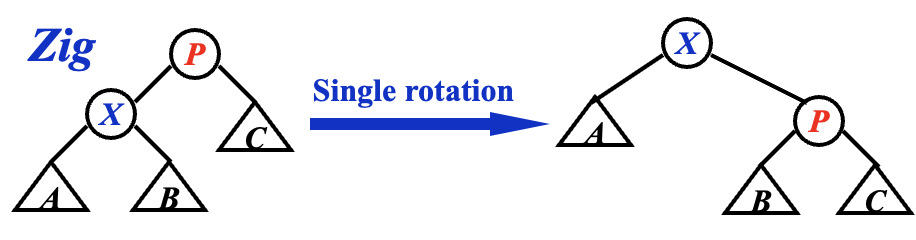\begin{aligned} {\hat{c}_{\text{zig}}}_i &= {c_{\text{zig}}}_i + \Phi_{i}(X) - \Phi_{i-1}(P) \\ &= 1 + Rank_{i}(X) - Rank_{i-1}(X) + Rank_{i}(P) - Rank_{i-1}(P) \end{aligned}

\left\{ \begin{aligned} & Rank_{i}(X) - Rank_{i-1}(X) \geq 0\\ & Rank_{i}(P) - Rank_{i-1}(P) \leq 0 \end{aligned} \right.

\begin{aligned} {\hat{c}_{\text{zig}}}_i &= 1 + \underbrace{Rank_{i}(X) - Rank_{i-1}(X)}_{\geq 0} + \underbrace{Rank_{i}(P) - Rank_{i-1}(P)}_{\leq 0} \\ &\leq 1 + Rank_{i}(X) - Rank_{i-1}(X) \end{aligned}

\begin{aligned} {\hat{c}_{\text{zig}}}_i &= 1 + Rank_{i}(P) - Rank_{i-1}(X) + \underbrace{Rank_{i}(X) - Rank_{i-1}(P)}_{= 0} \\ &= 1 + Rank_{i}(P) - Rank_{i-1}(X) \end{aligned}

$\hat{c}_{\text{rotate }x\text{ to root}} = \hat{c}_{\text{zig}} + \sum{ {\hat{c}_{\text{zig-zag}}}_i } + \sum{ {\hat{c}_{\text{zig-zig}}}_i }$

#### zig-zag¶

Amortized Analysis for Zig-Zag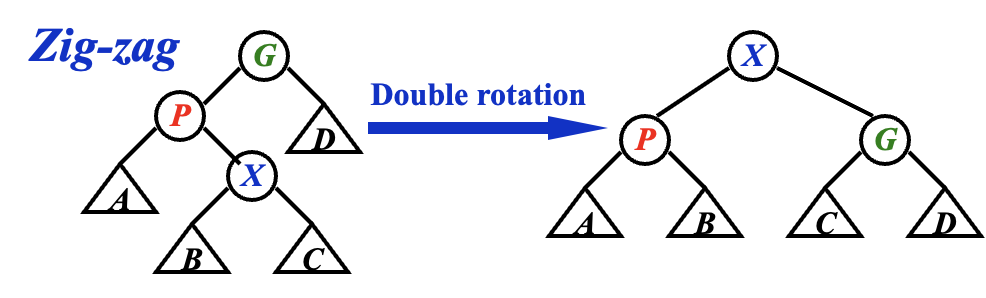\begin{aligned} {\hat{c}_{\text{zig-zag}}}_i &= {c_{\text{zig-zag}}}_i + \Phi_{i}(X)- \Phi_{i-1}(G) \\ &= 2 + Rank_{i}(X) - Rank_{i-1}(X) + Rank_{i}(P) - Rank_{i-1}(P) + Rank_{i}(G) - Rank_{i-1}(G) \end{aligned}

Lemma 1

\begin{aligned} \log{a} + \log{b} &= 2\left( \frac{\log{a} + \log{b}}{2} \right) \\ &\leq 2\left( \log{\frac{a+b}{2}} \right) \\ &= 2\left( \log{(a+b)} - \log{2} \right) \\ &= 2 \log{(a+b)} - 2 \\ \end{aligned}

\begin{aligned} Rank_{i}(P) + Rank_{i}(G) &= \log{Size_{i}(P)} + \log{Size_{i}(G)} \\ &\leq 2\log{\left(Size_{i}(P)+Size_{i}(G)\right)} - 2 & \text{(Lemma 1)}\\ &\leq 2\log{\left(Size_{i}(P)+Size_{i}(G)+1\right)} - 2 \\ &\leq 2\log{Size_{i}(X)} - 2 \\ &\leq 2Rank_{i}(X) - 2 \\ \end{aligned}

\left\{ \begin{aligned} Rank_{i}(X) &= Rank_{i-1}(G) \\ Rank_{i}(P) + Rank_{i}(G) &\leq 2Rank_{i}(X) - 2 \\ Rank_{i-1}(P) + Rank_{i-1}(X) &\geq 2Rank_{i-1}(X) \end{aligned} \right.

\begin{aligned} {\hat{c}_{\text{zig-zag}}}_i &= 2 + Rank_{i}(X) - Rank_{i-1}(X) + Rank_{i}(P) - Rank_{i-1}(P) + Rank_{i}(G) - Rank_{i-1}(G) \\ &= 2 + \left( Rank_{i}(X) - Rank_{i-1}(G)\right ) + \left( Rank_{i}(P) + Rank_{i}(G) \right) - \left( Rank_{i-1}(P) + Rank_{i-1}(X) \right) \\ &\leq 2 + 0 + 2Rank_{i}(X) - 2 + 2Rank_{i-1}(X) \\ &= 2\left(Rank_{i}(X) - Rank_{i-1}(X)\right) \end{aligned}

#### zig-zig¶

Amortized Analysis for Zig-Zig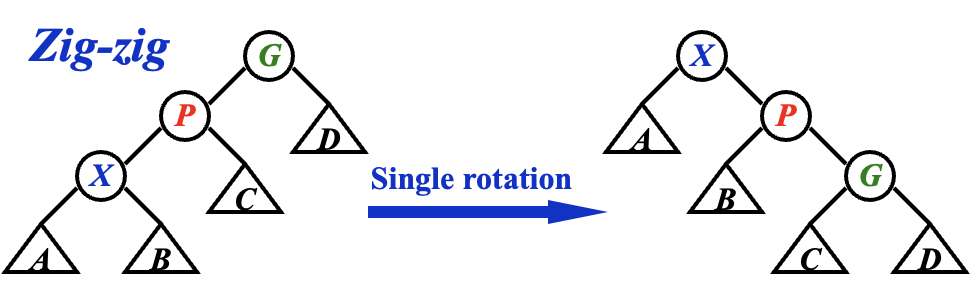\begin{aligned} {\hat{c}_{\text{zig-zig}}}_i &= {c_{\text{zig-zig}}}_i + \Phi_{i}(X)- \Phi_{i-1}(G) \\ &= 2 + Rank_{i}(X) - Rank_{i-1}(X) + Rank_{i}(P) - Rank_{i-1}(P) + Rank_{i}(G) - Rank_{i-1}(G) \end{aligned}

\begin{aligned} Rank_{i}(G) + Rank_{i-1}(X) &\leq 2\log{\left( \underbrace{Size_{i}(G)}_{\mathbf{C}+\mathbf{D}+G} + \underbrace{Size_{i-1}(X)}_{\mathbf{A}+\mathbf{B}+X} \right)} - 2 & \text{(Lemma 1)} \\ &\leq 2\log{\left( Size_{i}(G) + Size_{i-1}(X) + 1 \right)} - 2 & \\ &= 2\log{\left( Size_{i}(X) \right)} - 2 \\ &= 2Rank_{i}(X) - 2 \\ Rank_{i}(G) &\leq 2Rank_{i}(X) - Rank_{i-1}(X) - 2 \end{aligned}

\left\{ \begin{aligned} Rank_{i}(X) &= Rank_{i-1}(G) \\ Rank_{i}(G) &\leq 2Rank_{i}(X) - Rank_{i-1}(X) - 2 \\ Rank_{i}(P) &\leq Rank_{i}(X) \\ Rank_{i-1}(P) &\geq Rank_{i-1}(X) \end{aligned} \right.

\begin{aligned} {\hat{c}_{\text{zig-zig}}}_i &= 2 + Rank_{i}(X) - Rank_{i-1}(X) + Rank_{i}(P) - Rank_{i-1}(P) + Rank_{i}(G) - Rank_{i-1}(G) \\ &= 2 + \left( Rank_{i}(X) - Rank_{i-1}(G) \right) + Rank_{i}(G) + Rank_{i}(P) - Rank_{i-1}(P) - Rank_{i-1}(X) \\ &\leq 2 + 0 + \left( 2Rank_{i}(X) - Rank_{i-1}(X) - 2 \right) + Rank_{i}(X) - Rank_{i-1}(X) - Rank_{i-1}(X) \\ &= 3\left( Rank_{i}(X) - Rank_{i-1}(X) \right) \end{aligned}

😩 至此，我们总算是把牛鬼蛇神的 zig-zig 的证明也给搞定了。

#### 结论¶

Amortized Analysis for Splay

\begin{aligned} {\hat{c}_{\text{zig}}}_i &\leq 1 + Rank_{i}(X) - Rank_{i-1}(X) \\ {\hat{c}_{\text{zig-zag}}}_i &\leq 2\left(Rank_{i}(X) - Rank_{i-1}(X)\right) \\ {\hat{c}_{\text{zig-zig}}}_i &\leq 3\left(Rank_{i}(X) - Rank_{i-1}(X)\right) \end{aligned}

\begin{aligned} {\hat{c}_{\text{zig}}}_i &\leq 1 + 3\left( Rank_{i}(X) - Rank_{i-1}(X) \right) \\ {\hat{c}_{\text{zig-zag}}}_i &\leq 3\left( Rank_{i}(X) - Rank_{i-1}(X) \right) \\ {\hat{c}_{\text{zig-zig}}}_i &\leq 3\left( Rank_{i}(X) - Rank_{i-1}(X) \right) \end{aligned}

\begin{aligned} \hat{c}_{\text{rotate }x\text{ to root}} &= \hat{c}_{\text{zig}} + \sum{ {\hat{c}_{\text{zig-zag}}}_i } + \sum{ {\hat{c}_{\text{zig-zig}}}_i } \\ &= O(1) + 3\left( Rank_{N}(X) - Rank_{0}(X) \right) \\ &= O(\log{N}) \end{aligned}